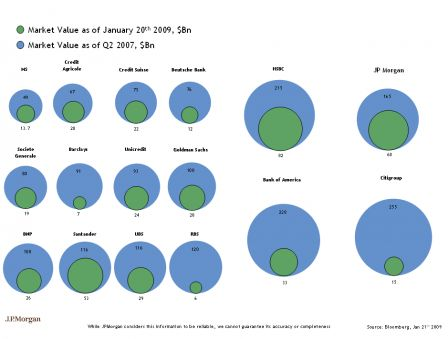Here is a proposed alternative to this bubble inferno pointed out in the revolutions blogand the R code behind it (here is the data). This is now item 150 in the graph gallery
```   1
2 ### read the data
3 d <- read.csv( "data.txt" )
4 d\$bank <- ordered( d\$bank, levels = d\$bank )
5
6 ### load lattice and grid
7 require( lattice )
8
9 ### setup the key
10 k <- simpleKey( c( "Q2 2007",  "January 20th 2009" ) )
11 k\$points\$fill <- c("lightblue", "lightgreen")
12 k\$points\$pch <- 21
13 k\$points\$col <- "black"
14 k\$points\$cex <- 1
15
16 ### create the plot
17 dotplot( bank ~ MV2007 + MV2009 , data = d, horiz = T,
18     par.settings = list(
19         superpose.symbol = list(
20             pch = 21,
21             fill = c( "lightblue", "lightgreen"),
22             cex = 4,
23             col = "black"
24         )
25      ) , xlab = "Market value (\$Bn)", key = k,
26      panel = function(x, y, ...){
27        panel.dotplot( x, y, ... )
28        grid.text(
29             unit( x, "native") , unit( y, "native") ,
30             label = x, gp = gpar( cex = .7 ) )
31      } )
```